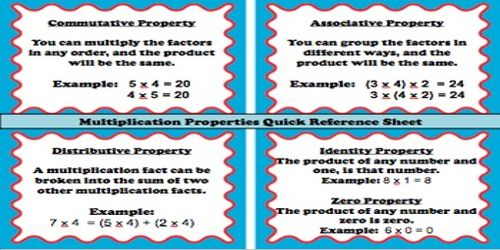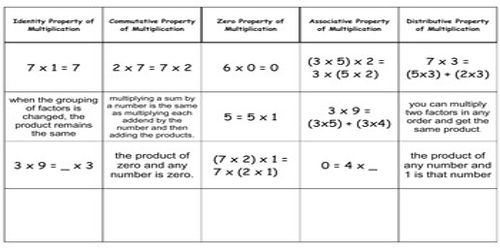Mathematic

# Multiplication PropertiesMultiplication Properties

For a property with such a long name, it’s really a simple math law. The multiplicative identity property states that any time you multiply a number by 1, the result, or product, is that original number.

To write out this property using variables, we can say that n * 1 = n. It doesn’t matter if n equals one, one million or 3.566879. The property always hold true. Therefore:

• 2 * 1 = 2
• 56 * 1 = 56
• 100,000,000,000 * 1 = 100,000,000,000There are four properties involving multiplication that will help make problems easier to solve. They are the commutative, associative, multiplicative identity and distributive properties.

Commutative property: When two numbers are multiplied together, the product is the same regardless of the order of the multiplicands. For example: 4 * 2 = 2 * 4. Changing the order of factors does not change the product.

Associative Property: Changing the grouping of factors does not change the product. When three or more numbers are multiplied, the product is the same regardless of the grouping of the factors. For example (2 * 3) * 4 = 2 * (3 * 4)

Multiplicative Identity Property: The product of 111 and any number is that number. The product of any number and one is that number. For example 5 * 1 = 5.

Distributive property: One factor in a multiplication problem can be broken apart to find partial products. He sum of the partial products is the product of the two factors. The sum of two numbers times a third number is equal to the sum of each addend times the third number. For example 4 * (6 + 3) = 4*6 + 4*3

Zero Property of Multiplication: When any number is multiplied by 0, the product is 0. 8 x 0 = 0.

Information Source: